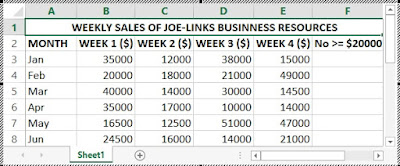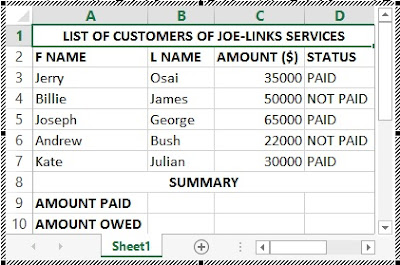## Friday, 4 November 2016

The SUMIF, COUNTIF and CONCATENATE functions are Excel’s built-in functions. These functions can also be applied practically to business systems.
This is the Part 3 of chapter 2 of Excel tutorial. You are advises to read the first two parts:

In this part 3 of chapter to of Excel tutorial, I will explicitly explain the hidden details of SUNIF, COUNTIF, CONCATENATE with practical illustrations.

### COUNTIF FUNCTION

This is one of MS-Excel’s Powerful Statistical functions that counts the total number of cells that met a specified condition within a specified range of cells.

The syntax for the COUNTIF function is =COUNTIF(Range, Criteria) press Enter.

The function takes two arguments:
1. Range: This is the range of cells excel will count if the meet the given condition.

2. Criteria: This is the specified condition a cell must match before Excel can count it.

## Practical Illustration of the Excel COUNTIF Function

The table shown in the worksheet below is used to for recording the weekly sales of Joe-Links Business Resources for the first six weeks of the year 2016. Use the COUNTIF function to count the number of weeks for each month where the amount of sales made is not less than \$20000.COUNTIF function – Table 1

### SOLUTION TO THE COUNTIF QUESTION

1. Prepare a worksheet like the one shown in the figure above.

2. Place your cell pointer in cell F3 and type the following syntax =COUNTIF(B3:E3,”>=20000”) press Enter. Take note of the quote in the syntax. It is mandatory.

B3:E5 is the range of cells for the four weeks of the month January.
“>=20000” is the criteria a cell in the range must meet before Excel counts it.

Don't copy these syntaxes. Type them yourself.

3. Use the auto fill handle to fill the subsequent spaces in the column.

The figure below contains the completed table of the question above.## SUMIF FUNCTION

This is another MS-Excel’s powerful function that adds the value in the cells within a specified range that met a specified criterion with respect to the values of another specified range. The SUMIF function is similar to the COUNTIF function, but requires two ranges of data to be specified.

The syntax for the SUMIF function is =SUMIF(Range,Criteria,Sum_range) press Enter.

The function takes three arguments. The first two – The Range and Criteria are the same as in the COUNTIF function. The third argument is the Sum_range which is the range of cells containing the values you wish to sum with respect to the set criteria.

## Practical Illustration of the Excel SUMIF Function

The table contained in the figure below shows a list of individuals who ordered goods from Joe-Links Services and their payment status. In the Status column, PAID indicates individuals that paid for the goods they received. While NOT PAID indicates individuals that have not. Using the SUMIF function, calculate the Total Amount Paid and the Total Amount Owed by the customers.### SOLUTION TO THE SUMIF QUESTION

1. Prepare your worksheet like the one shown in the figure above.
2. Type in the following syntax in cell B9 =SUMIF(D3:D7,”PAID”,C3:C7) press Enter.
3. Type in the following syntax in cell B10 =SUMIF(D3:D7,”NOT PAID”,C3:C7) press Enter.
4. D3:D7 is the range of cells you want to check for PAID and NOT PAID values i.e. the range argument.

PAID and NOT PAID are the criteria in which the sum is based i.e. the criteria argument.
C3:c7  is the range of cells that contain the value you want add based on the specified criteria i.e. the sm_range argument.

Your worksheet should now look like the one in the figure below.## CONCATENATE FUNCTION WITH ILLUSTRATION

This is an Excel built-in function used to join two or more text items together.

The syntax for the CONCATENATE function looks like this: =CONCATENATE(TEXT1,TEXT2,TEXT3) press Enter.

The function takes up to 255 text items. A comma is used to separate each text item. If you actually a comma or a space or any special character, you need to treat it as a text. If the item is a text, you enclose it in double quote. A comma, space or any special character is treated as a text.
If the item is a number, or a formula, you don’t need to enclose it in a double quote.

For example, the output of the CONCATENATE function:
=CONCATENATE(“Keyboard”,”=”,2,” “,“Mouse”,”=”,3,” “,“Monitor”,”=”,4) is:
Keyboard=2 Mouse=3 Monitor=4

This is the end of the part 3 of chapter 2.
Now go over to Part 4: HOW TO CALCULATE YOUR CGPA USING MS-EXCEL